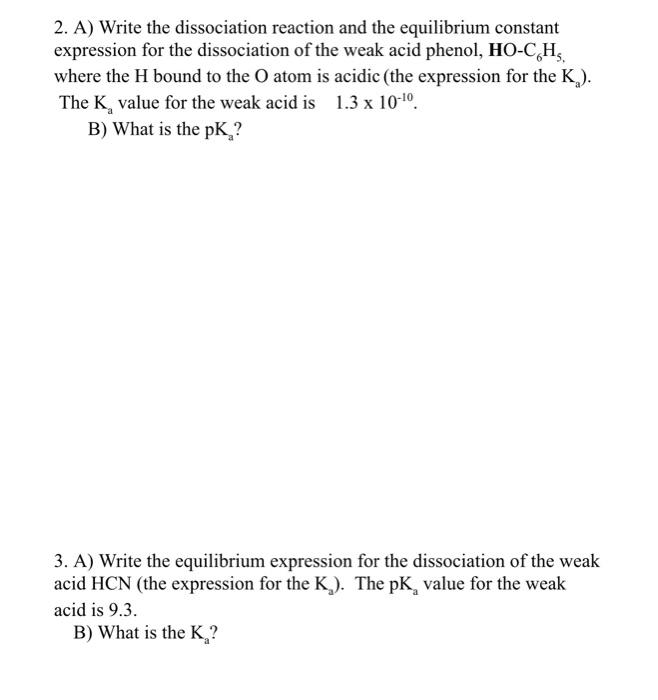Home / Expert Answers / Chemistry / 2-a-write-the-dissociation-reaction-and-the-equilibrium-constant-expression-for-the-dissociation-pa872

# (Solved): 2. A) Write the dissociation reaction and the equilibrium constant expression for the dissociation ...2. A) Write the dissociation reaction and the equilibrium constant expression for the dissociation of the weak acid phenol, , where the bound to the atom is acidic (the expression for the ). The value for the weak acid is . B) What is the ? 3. A) Write the equilibrium expression for the dissociation of the weak acid (the expression for the ). The value for the weak acid is 9.3 . B) What is the ?

We have an Answer from Expert